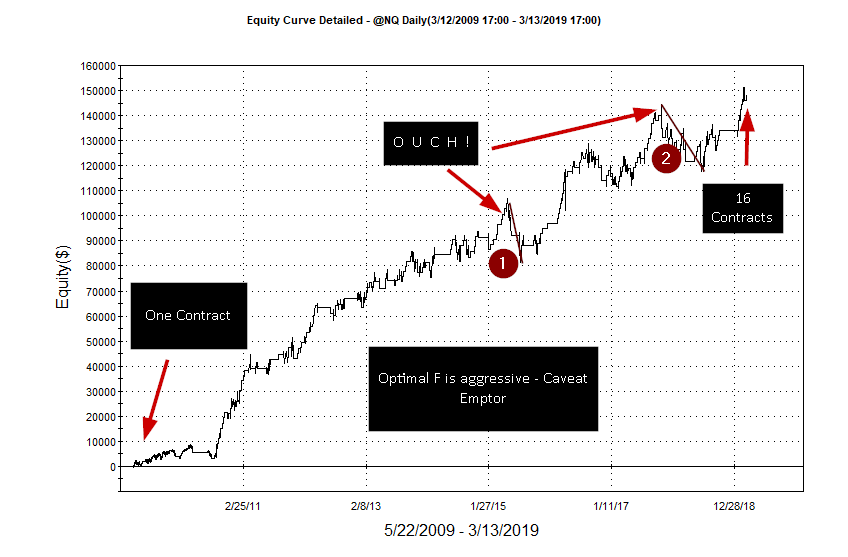# Calculating Position Size with Optimal F

I had a reader of the blog ask how to use Optimal F.  That was really a great question.  A few posts back I provided the OptimalFGeo function but didn’t demonstrate on how to use it for allocation purposes.  In this post, I will do just that.

## I Have Optimal F – Now What?

From Ralph Vince’s book, “Portfolio Management Formulas”, he states: “Once the highest f is found, it can readily be turned into a dollar amount by dividing the biggest loss by the negative optimal f.  For example, if our biggest loss is \$100 and our optimal f is 0.25, then -\$100/ 0.25 = \$400.  In other words, we should bet 1 unit for every \$400 we have in our stake.”

### Convert Optimal F to dollars and then to number of shares

In my example strategy, I start out with an initial capital of \$50,000 and allow reinvestment of profit or loss.  The protective stop is set as 3 X ATR(10).  A fixed \$2000 profit objective is also utilized.  The conversion form Optimal F to position size is illustrated by the following lines of code:

``//keep track of biggest lossbiggestLoss = minList(positionProfit(1),biggestLoss);//calculate the Optimal F with last 10 trades.OptF = OptimalFGeo(10);//reinvest profit or lossrisk\$ = initCapital\$ + netProfit;//convert Optimal F to \$\$\$if OptF <> 0 then numShares = risk\$ / (biggestLoss / (-1*OptF));``
Code snippet - Optimal F to Position Size
1. Keep track of biggest loss
2. Calculate optimal F with OptimalFGeo function – minimum 10 trades
3. Calculate Risk\$ by adding InitCapital to current NetProfit (Easylanguage keyword)
4. Calculate position size by dividing Risk\$  by the quotient of biggest loss and (-1) Optimal F

I applied the Optimal F position sizing to a simple mean reversion algorithm where you buy on a break out in the direction of the 50-day moving average after a lower low occurs.

Code listing:

``vars: numShares(0),initCapital\$(50000),biggestLoss(0),OptF(0),risk\$(0);//keep track of biggest lossbiggestLoss = minList(positionProfit(1),biggestLoss);//calculate the Optimal F with last 10 trades.OptF = OptimalFGeo(10);//reinvest profit or lossrisk\$ = initCapital\$ + netProfit;//convert Optimal F to \$\$\$if OptF <> 0 then numShares = risk\$ / (biggestLoss / (-1*OptF));numShares =  maxList(1,numShares);//if Optf <> 0 then print(d," ",t," ",risk\$ / (biggestLoss / (-1*OptF))," ",biggestLoss," ",optF);if c > average(c,50) and low < low then Buy numShares shares next bar at open + .25* range stop;setStopPosition;setProfitTarget(2000);setStopLoss(3*avgTrueRange(10)*bigPointValue);``
Strategy Using Optimal F

I have included the results below.  At one time during the testing the number of contracts jumped up to 23.  That is 23 mini Nasdaq futures (\$20 * 7,300) * 23.  That’s a lot of leverage and risk.  Optimal doesn’t  always mean the best risk mitigation.Please let me know if you find any errors in the code or in the logic.

Here is the ELD that incorporates the Strategy and the Function.USINGOPTIMALF

## 4 thoughts on “Calculating Position Size with Optimal F”

1.steve says:

I enjoyed this George. Any chance you\’ll take a stab at Mr. Vince\’s Leverage Space Portfolio next? If so, how about in Visual Basic?

1.George Pruitt says:

Hi Steve – I might do this in Python. Wouldn’t be difficult to convert to VB I wouldn’t think.

2.Sam says:

hi
my English is bad.But,I want to say that there\’s something wrong in the logic. In the book of Ralph Vince’s Book, the Optimal f means tracing the all the passed trades ,by looping from 1 to 0.01 the value f to find the
highest TWR.
to use the optimal f ,the program must first Simulate lots of trades. after that , we might use optimal f to Calculate the best share number into the following trades under an Hypothetical condition Winning probability is fixed.

1.George Pruitt says:

Hi Sam – thanks for commenting on the post. Take a look at the function that does the actual calculation.
https://georgepruitt.com/easylanguage-code-for-optimal-f-multi-charts-and-vba-too/